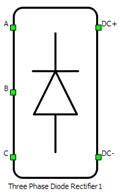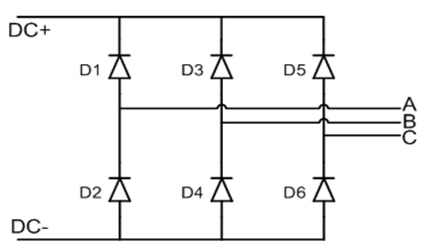# Three Phase Diode Rectifier

Description of the Three Phase Diode Rectifier component in Schematic Editor

A block diagram and input parameters for a three-phase diode rectifier block are given in Table 1.

Table 1. Three Phase Diode Rectifier component in the Schematic Editor core library
component component dialog window component parametersThree Phase Diode Rectifier• PESB _Optimization
Weight = 3

A schematic block diagram of a three-phase inverter is given in Figure 1 with its corresponding switch arrangement and naming, and internal voltage and current measurements. Internal measurements cannot be selected as analog outputs (on the HIL hardware) via the HIL SCADA.Figure 1. A schematic block diagram of a Three Phase Diode Rectifier block with corresponding diode naming

## PESB Optimization

The PESB Optimization option is available in certain converter models. When PESB Optimization is enabled, all converter's short circuit state space modes will be merged and treated as the same state space mode. For example, if one converter leg within the three phase converter is short circuited and PESB Optimization is enabled, all of the legs within the three phase converter will also be short circuited. This simplification for short circuit modeling can save a significant amount of matrix memory.

## Losses calculation

When the Losses calculation property is enabled, the component will calculate switching and conduction power losses for all switching elements (Diodes). Switching power losses are calculated as a function of current, voltage, and temperature using 3D Look-up tables (LUTs). Also, 2D input for losses is supported. When a 2D losses table is inserted, it assumes only current and temperature dependance. Conduction power losses can be defined as a function of current and temperature using Vd Look-up tables. These LUTs can be either 1D or 2D. If the LUT is a 1D table, the forward voltage drop depends only on current. If the LUT is a 2D table, the forward voltage drop dependence on the junction temperature is included.

Import options and an explanation of how to correctly fill in all the necessary power loss parameters are described in the Importing power losses data section.

Note: An interactive overview of how you can incorporate power loss calculation in your model is available as part of the HIL for Power Electronics course on HIL Academy, as well as in the video Knowledgebase.
Input/output power losses ports receive/generate vectors of six elements. The first element (index 0) is the upper diode in phase A, the second element (index 1) is the bottom diode in phase A. The 3rd and 4th elements follow this same pattern for phase B, as do the 5th and 6th elements for phase C. Available mask properties are:
• Current values - Switching elements current axis [A]
• Voltage values - Switching elements voltage axis [V]
• Temp values - Switching elements temperature axis [°C]
• Vd table - Diode forward voltage drop, f(I,T) [V]
• Ed off table - Diode switching OFF losses, output energy, f(I, V, T) [J]

## Temperatures calculation

When the Temperatures calculation property is enabled, the component calculates the combined power losses (P_loss) and junction temperatures (T_junctions) for all switching elements (Diodes). Combined power losses represent the sum of the calculated switching and conduction losses transferred through an internally generated Thermal network component. The internally generated Thermal network component also calculates the junction temperatures from power losses, input case temperatures, and provided thermal model parameters. Input/output ports for temperature calculation are vectors of six elements and they are indexed in the same way as explained in the Losses calculation section.

Note: An interactive overview of how you can incorporate temperature calculation in your model is available as part of the HIL for Power Electronics course on HIL Academy, as well as in the video Knowledgebase.